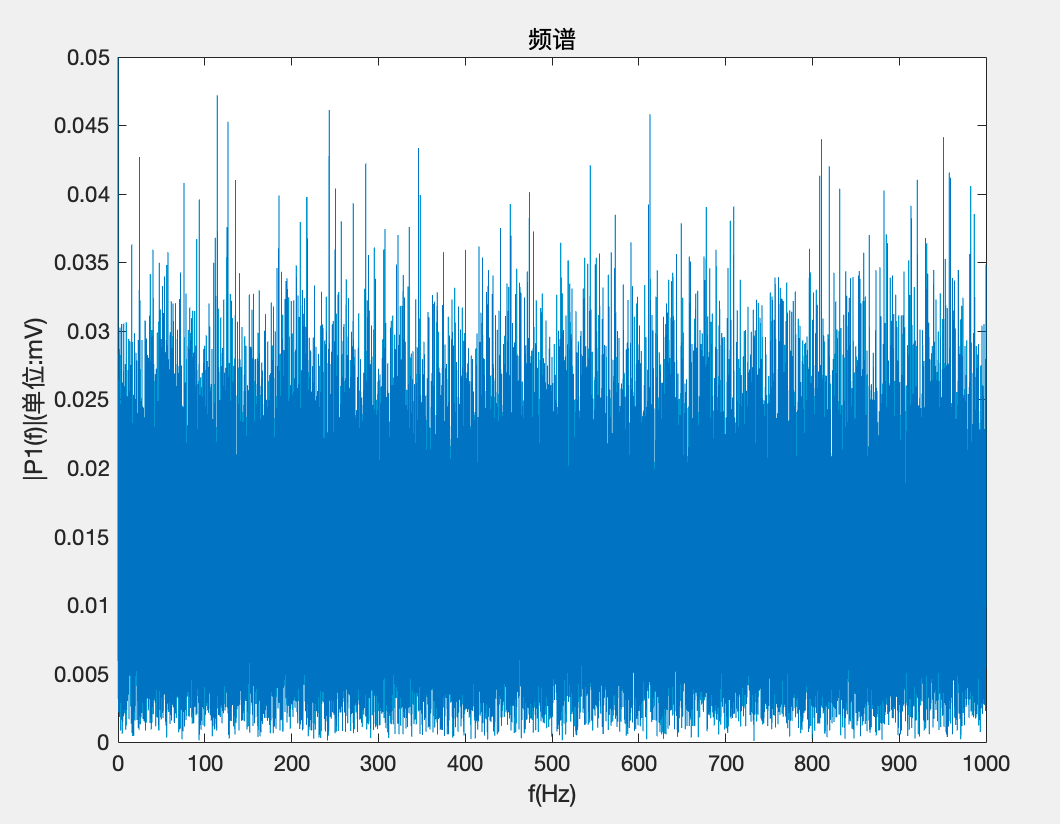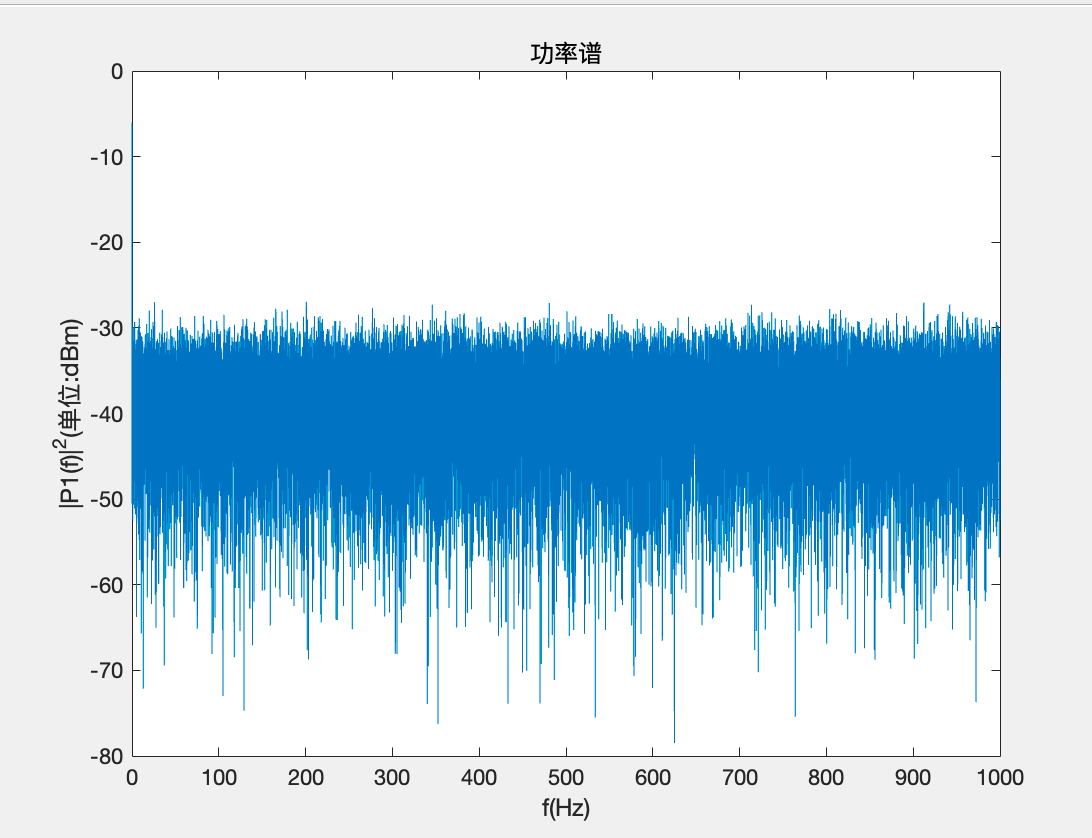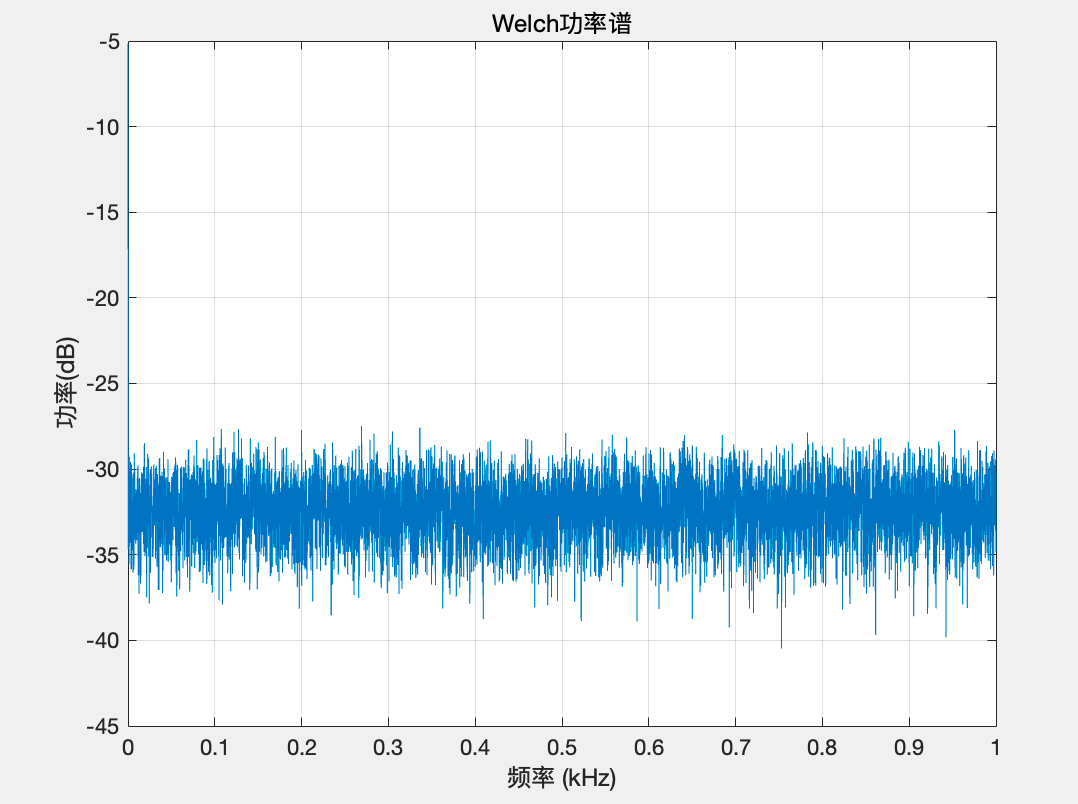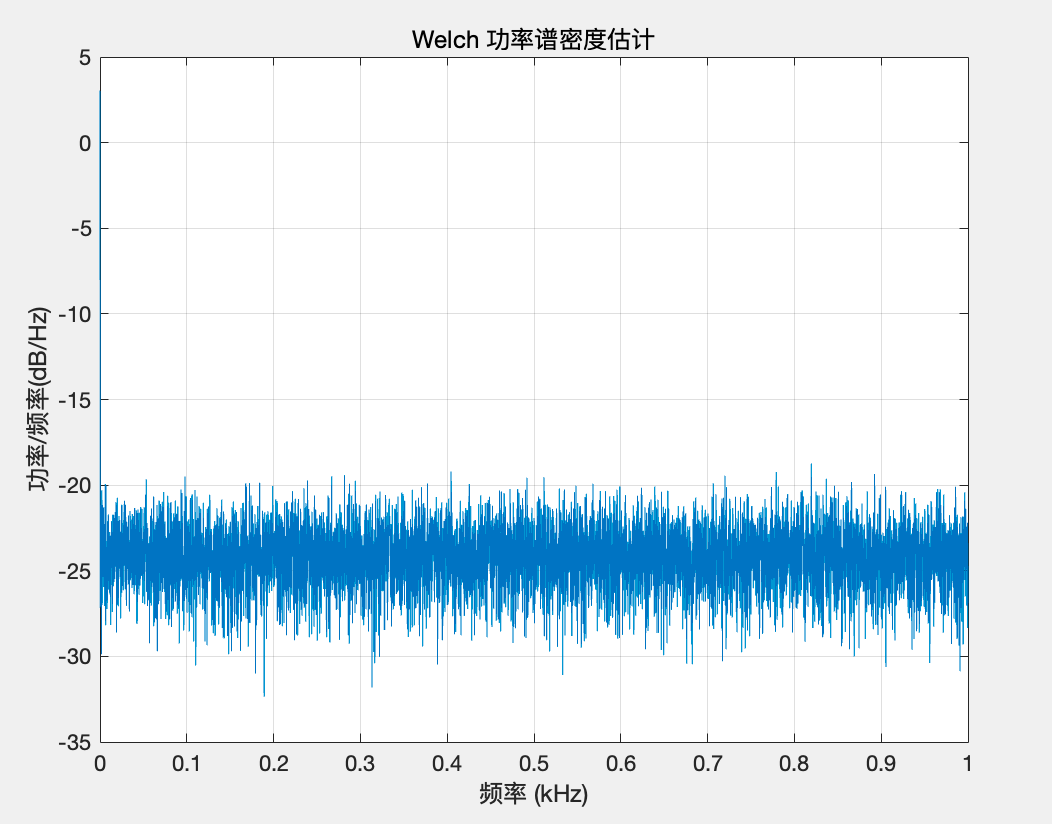Sorry, your browser cannot access this site

MengFanjun的博客

# 介绍

## 频谱

频谱是指某个信号在频域上的表示，即它在不同频率下的成分及其相对强度。在信号处理中，频谱常用于分析信号的频率内容和频率分布，可以用于音频、视频、无线通信等领域的分析和处理。频谱通常用图形方式表示，其中横轴表示频率，纵轴表示信号在该频率上的强度或功率，可以是线性或对数坐标。

## 功率谱

功率谱表示单位频带内信号功率随频率的变化情况,单位是W，即它在不同频率下的功率或能量分布情况。与频谱类似，功率谱也是用于分析信号频率内容和频率分布的一种工具，但它考虑的是信号在不同频率下的功率或能量分布，因此可以更直观地描述信号的能量分布情况。通常，功率谱的横坐标是频率，纵坐标是功率或能量密度，可以是线性或对数坐标。功率谱在很多领域都有广泛的应用，比如无线通信、音频信号处理、图像处理等。

## 功率谱密度

功率谱密度表示单位时间和单位频带内信号能量随频率的分布情况，单位是能量除时间除频带，通常用于描述信号在连续频域上的功率分布情况。与功率谱类似，功率谱密度也是描述信号在不同频率下的功率或能量分布情况，但它考虑的是单位频率范围内的功率或能量分布情况，因此它可以更精确地描述信号的功率分布情况，尤其是对于带宽非常宽的信号而言。功率谱密度通常用于分析连续时间信号或随机信号的频率特性，比如噪声、振动、电磁干扰等。功率谱密度的单位通常是功率或能量密度除以频率单位，例如瓦特/赫兹（W/Hz）或焦耳/赫兹（J/Hz）。

# 由电路中的功率想到信号功率

$电路中的功率:P=\frac{V^2}{R}=I^2R$

$R=1\Omega$

$电路中的功率:P=V^2=I^2$

## 计算信号能量和能量谱密度

$G(f)=\left | s(f) \right |^2,J/HZ$

## 计算信号功率和功率谱密度

$E_{T}=\int_{-\frac{T}{2} }^{\frac{T}{2} } s_{T}(t)^2 dt=\int_{-\infty }^{\infty }\left | S_{T}(f)^2 \right | df$

$P(f)=\lim_{T \to \infty} \frac{1}{T}\left |S(f) \right |^2$ P(f)就是功率谱密度 功率信号的功率P就是： $P=\lim_{T \to \infty} \int \left |S(f) \right |^2df=\int_{-\infty }^{\infty } P(f) df$ P存在，即称为功率信号 由上面的式子可知：

# MATLAB画一个随机信号的频谱

## 做傅立叶变换并画图因为没有进行任何的滤波等操作，所以里面的直流分量、噪声等影响因素都存在，这就是一个很完全的原始信号。 ## MATLAB画这个随机信号的功率谱 功率实际上就是能量的平方。 为了便于观察功率谱的变化，我们对横坐标的功率化成了dBm $dBm=10log_{10}\frac{P}{1mW}$## MATLAB画这个随机信号的功率谱(另一种方法)## MATLAB画这个随机信号的功率谱密度功率谱密度纵轴的单位变成了(db/Hz)，表示每一个Hz有多少dB。 跟功率谱图像做对比，可以观察到，幅度较大的频率分量被保留了下来。

### pwelch函数含义

pwelch是Matlab中用于计算功率谱密度的函数。它的基本语法是：